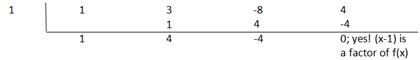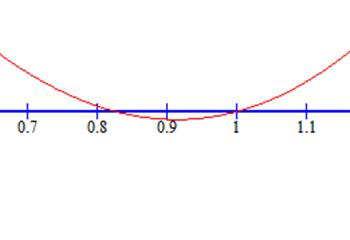### Sample Problem

Find all real solutions of the polynomial equation x3+3x2-8x+4=0

#### Solution

Using the Rational Zero Test, we know that all the possible rational solutions are factors of 4:

±2, ±4, ±1After checking the graph, we see the only plausible rational factor is 1.x3+3x2-8x+4=(x-1)(x2+4x-4)

Using the Quadratic Formula, we can then factor x2+4x-4=0 where a=1, b=4, c=-4Good thing we checked the factorization otherwise we would not have caught the -2+2√2 solution, since it is so close so x=1 (also a factor).

.So all the real zeros of the function are: x=1, -2-2√2, -2+2√2.